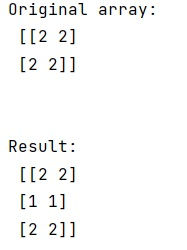# How to insert a row at a specific location in a 2d array in NumPy?

Given a 2D NumPy array, we have to insert a row at a specific location in it.
Submitted by Pranit Sharma, on March 09, 2023

## NumPy: Inserting a row at a specific location in 2D array

Suppose that we are given a 2D NumPy array like [[2,2],[2,2]] and we need to insert a row at a specific location in this array (say after 1st row).

To insert a row at a specific location, we can simply use numpy.insert() function. We will first create another numpy 1D array (the row that needs to be inserted) and pass this array at index 1 (so that it is inserted after index 1).

Let us understand with the help of an example,

## Python code to insert a row at a specific location in a 2d array in NumPy

```# Import numpy
import numpy as np

# Creating a numpy array
arr = np.array([[2,2],[2,2]])

# Display Original array
print("Original array:\n",arr,"\n\n")

# Creating another array
arr2 = np.array([1,1])

# Inserting arr2 after 1st row in arr
res = np.insert(arr,1,arr2,0)

# Display result
print("Result:\n",res)
```

### OutputLanguages: » C » C++ » C++ STL » Java » Data Structure » C#.Net » Android » Kotlin » SQL
Web Technologies: » PHP » Python » JavaScript » CSS » Ajax » Node.js » Web programming/HTML
Solved programs: » C » C++ » DS » Java » C#
Aptitude que. & ans.: » C » C++ » Java » DBMS
Interview que. & ans.: » C » Embedded C » Java » SEO » HR
CS Subjects: » CS Basics » O.S. » Networks » DBMS » Embedded Systems » Cloud Computing
» Machine learning » CS Organizations » Linux » DOS
More: » Articles » Puzzles » News/Updates